ML Aggarwal Class 6 Solutions for ICSE Maths Model Question Paper 5 acts as the best resource during your learning and helps you score well in your exams.

## ML Aggarwal Class 6 Solutions for ICSE Maths Model Question Paper 5

Choose the correct answer from the given four options (1-2):
Question 1.
The number of lines of symmetry of a protractor is
(a) 0
(b) 1
(c) 2
(d) unlimited
Solution:
1 (b)

Question 2.
If the perimeter of a regular pentagon is 60 cm, then its every side is
(a) 10 cm
(b) 12 cm
(c) 15 cm
(d) 20 cm
Solution: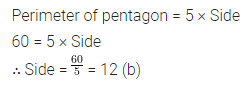Question 3.
If the perimeter of a square is 42 cm, then find its area.
Solution:Question 4.
Using a ruler and compass, construct an angle of 90°.
Solution:
Steps of Construction:Question 5.
On a squared paper, sketch a quadrilateral with exactly two lines of symmetry. Also, sketch the lines of symmetry.
Solution: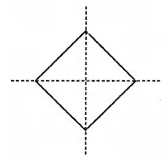Question 6.
If the area of a rectangular plot is 396 sq. m and its breadth is 18 m. Find the length of the plot and the cost of fencing it at the rate of ₹7.50 per metre.
Solution:Question 7.
Draw a line segment AB of length 6.4 cm. Take a point P on AB such that AP = 4.5 cm. Draw a perpendicular to AB at P. (use ruler and compass).
Solution: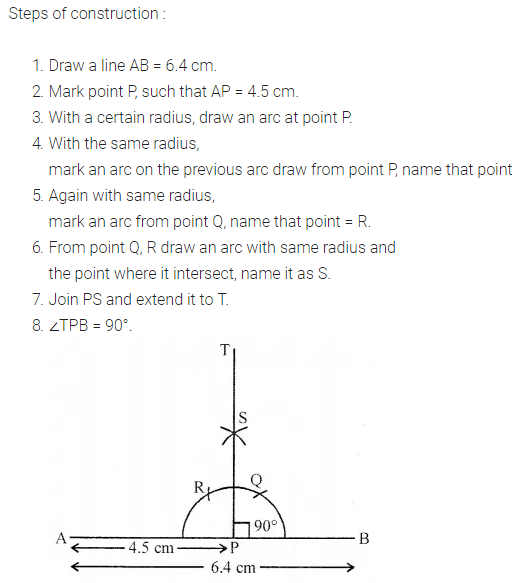Question 8.
Copy the given figure. How many lines of symmetry it has? Draw its all lines of symmetry.Solution: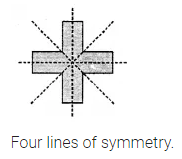Question 9.
In the given figure, all adjacent sides are at right angles. Find:
(i) the perimeter of the figure.
(ii) the area enclosed by the figure.Solution:Question 10.
Copy the given figure on a squared paper and complete the figure such that the resultant figure is symmetrical about both the dotted lines.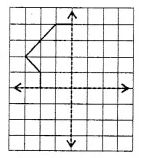Solution: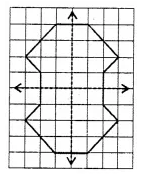https://ncertsolutionsguru.dreamwidth.org/
https://www.racked.com/users/NCERTSolutionsGuru
https://github.com/NCERTSolutionsGuru